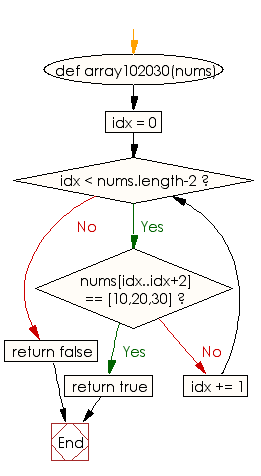﻿ Ruby Basic exercises: Check whether the sequence of numbers 10, 20, 30 appears anywhere in a given array of integers - w3resource# Ruby Basic Exercises: Check whether the sequence of numbers 10, 20, 30 appears anywhere in a given array of integers

## Ruby Basic: Exercise-43 with Solution

Write a Ruby program to check whether the sequence of numbers 10, 20, 30 appears anywhere in a given array of integers.

Ruby Code:

``````def array102030(nums)
idx = 0
while idx < nums.length-2
if nums[idx..idx+2] == [10,20,30]
return true
end
idx += 1
end
return false
end
print array102030([10, 20, 30, 40, 50]),"\n"
print array102030([0, 10, 20, 30, 90]),"\n"
print array102030([10, 20, 50, 30, 70])
``````

Output:

```true
true
false
```

Flowchart:Ruby Code Editor: